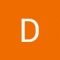Class Central is learner-supported. When you buy through links on our site, we may earn an affiliate commission.

Probabilistic Graphical Models 2: Inference

Overview

Class Central Tips
Probabilistic graphical models (PGMs) are a rich framework for encoding probability distributions over complex domains: joint (multivariate) distributions over large numbers of random variables that interact with each other. These representations sit at the intersection of statistics and computer science, relying on concepts from probability theory, graph algorithms, machine learning, and more. They are the basis for the state-of-the-art methods in a wide variety of applications, such as medical diagnosis, image understanding, speech recognition, natural language processing, and many, many more. They are also a foundational tool in formulating many machine learning problems.

This course is the second in a sequence of three. Following the first course, which focused on representation, this course addresses the question of probabilistic inference: how a PGM can be used to answer questions. Even though a PGM generally describes a very high dimensional distribution, its structure is designed so as to allow questions to be answered efficiently. The course presents both exact and approximate algorithms for different types of inference tasks, and discusses where each could best be applied. The (highly recommended) honors track contains two hands-on programming assignments, in which key routines of the most commonly used exact and approximate algorithms are implemented and applied to a real-world problem.

Syllabus

• Inference Overview
• This module provides a high-level overview of the main types of inference tasks typically encountered in graphical models: conditional probability queries, and finding the most likely assignment (MAP inference).
• Variable Elimination
• This module presents the simplest algorithm for exact inference in graphical models: variable elimination. We describe the algorithm, and analyze its complexity in terms of properties of the graph structure.
• Belief Propagation Algorithms
• This module describes an alternative view of exact inference in graphical models: that of message passing between clusters each of which encodes a factor over a subset of variables. This framework provides a basis for a variety of exact and approximate inference algorithms. We focus here on the basic framework and on its instantiation in the exact case of clique tree propagation. An optional lesson describes the loopy belief propagation (LBP) algorithm and its properties.
• MAP Algorithms
• This module describes algorithms for finding the most likely assignment for a distribution encoded as a PGM (a task known as MAP inference). We describe message passing algorithms, which are very similar to the algorithms for computing conditional probabilities, except that we need to also consider how to decode the results to construct a single assignment. In an optional module, we describe a few other algorithms that are able to use very different techniques by exploiting the combinatorial optimization nature of the MAP task.
• Sampling Methods
• In this module, we discuss a class of algorithms that uses random sampling to provide approximate answers to conditional probability queries. Most commonly used among these is the class of Markov Chain Monte Carlo (MCMC) algorithms, which includes the simple Gibbs sampling algorithm, as well as a family of methods known as Metropolis-Hastings.
• Inference in Temporal Models
• In this brief lesson, we discuss some of the complexities of applying some of the exact or approximate inference algorithms that we learned earlier in this course to dynamic Bayesian networks.
• Inference Summary
• This module summarizes some of the topics that we covered in this course and discusses tradeoffs between different algorithms. It also includes the course final exam.

Daphne Koller

Reviews

4.3 rating, based on 3 reviews

Start your review of Probabilistic Graphical Models 2: Inference

•Dmytro Aleksandrov
1

Dmytro Aleksandrov completed this course, spending 20 hours a week on it and found the course difficulty to be hard.

An immersing, challenging and time-consuming course, just as it's first part. Helped me improve understanding of ML foundations theory. Programming assignments may look a bit archaic, as I see that Matlab/Octave isn't gaining traction in ML community nowadays, but it's a language that's expressive enough, and in no way makes this course boring or worthless. Although it would be nice if was accompanied with assignments in Python or R or Scala.
• Tianpei Xie completed this course.

• Stephane Mysona
3

Stephane Mysona completed this course.Never Stop Learning!

Get personalized course recommendations, track subjects and courses with reminders, and more.